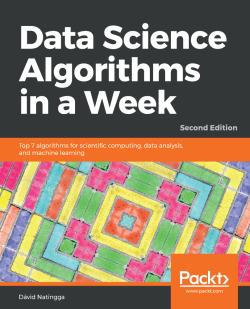# Data Science Algorithms in a Week - Second Edition4 (1 reviews total)
By Dávid Natingga
• \$33.99 eBook
• \$12.99 eBook + Subscription
What do you get with a Packt Subscription?

• Constantly updated with 100+ new titles each month
• Breadth and depth in over 1,000+ technologies

Machine learning applications are highly automated and self-modifying, and continue to improve over time with minimal human intervention, as they learn from the trained data. To address the complex nature of various real-world data problems, specialized machine learning algorithms have been developed. Through algorithmic and statistical analysis, these models can be leveraged to gain new knowledge from existing data as well.

Data Science Algorithms in a Week addresses all problems related to accurate and efficient data classification and prediction. Over the course of seven days, you will be introduced to seven algorithms, along with exercises that will help you understand different aspects of machine learning. You will see how to pre-cluster your data to optimize and classify it for large datasets. This book also guides you in predicting data based on existing trends in your dataset. This book covers algorithms such as k-nearest neighbors, Naive Bayes, decision trees, random forest, k-means, regression, and time-series analysis.

By the end of this book, you will understand how to choose machine learning algorithms for clustering, classification, and regression and know which is best suited for your problem

Publication date:
October 2018
Publisher
Packt
Pages
214
ISBN
9781789806076

## Chapter 1. Classification Using K-Nearest Neighbors

A nearest neighbor algorithm classifies a data instance based on its neighbors. The class of a data instance determined by the k-nearest neighbors algorithm is the class with the highest representation among the k-closest neighbors.

In this chapter, we will cover the following topics:

• How to implement the basics of the k-NN algorithm using the example of Mary and her temperature preferences
• How to choose a correctk value so that the algorithm can perform correctly and with the highest degree of accuracy using the example of a map of Italy
• How to rescale values and prepare them for the k-NN algorithm using the example of house preferences
• How to choose a good metric to measure distances between data points
• How to eliminate irrelevant dimensions in higher-dimensional space to ensure that the algorithm performs accurately using the text classification example

## Mary and her temperature preferences

As an example, if we know that our friend, Mary, feels cold when it is 10°C, but warm when it is 25°C, then in a room where it is 22°C, the nearest neighbor algorithm would guess that our friend would feel warm, because 22 is closer to 25 than to 10.

Suppose that we would like to know when Mary feels warm and when she feels cold, as in the previous example, but in addition, wind speed data is also available when Mary is asked whether she feels warm or cold:

 Temperature in °C Wind speed in km/h Mary's perception 10 0 Cold 25 0 Warm 15 5 Cold 20 3 Warm 18 7 Cold 20 10 Cold 22 5 Warm 24 6 Warm

We could represent the data in a graph, as follows: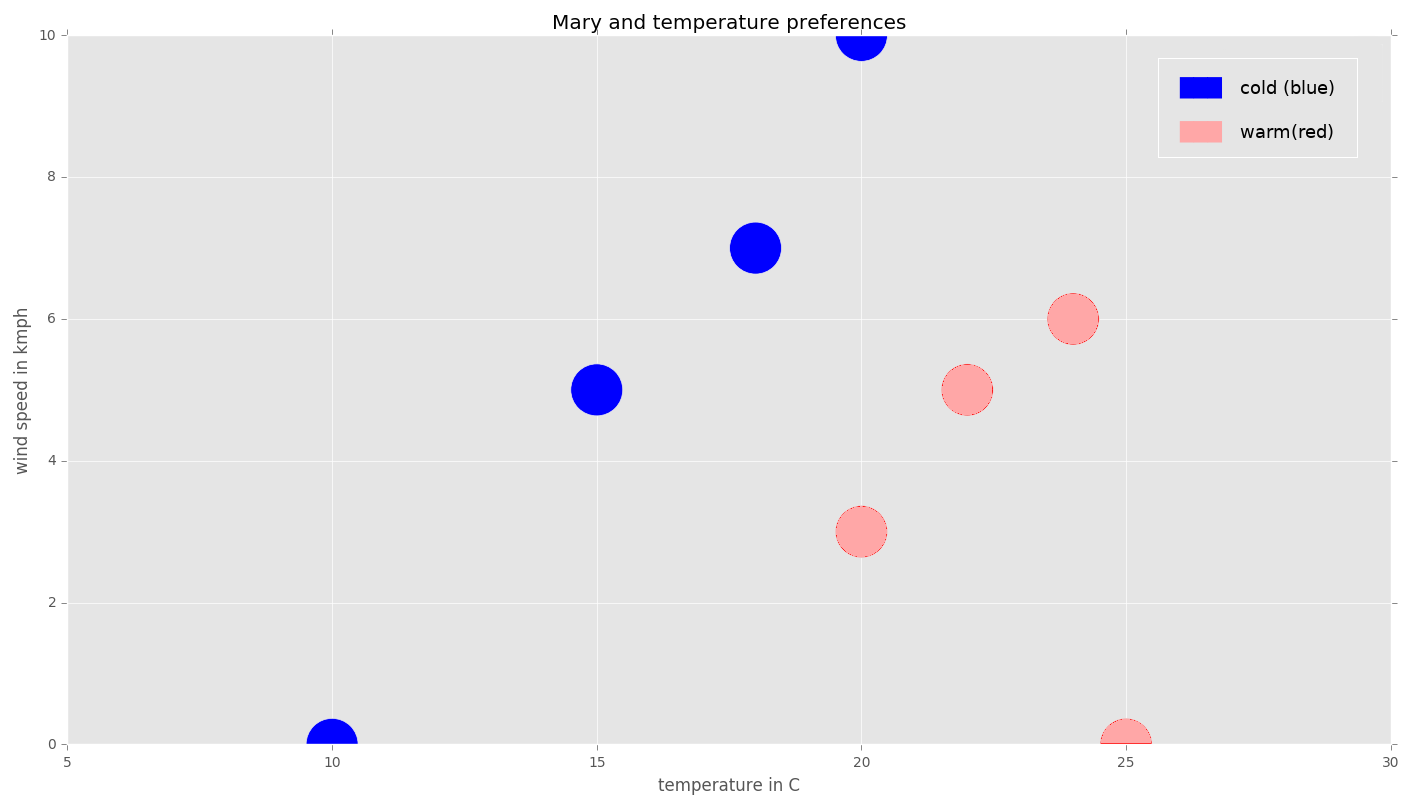Now, suppose we would like to find out how Mary feels when the temperature is 16°C with a wind speed of 3 km/h by using the 1-NN algorithm: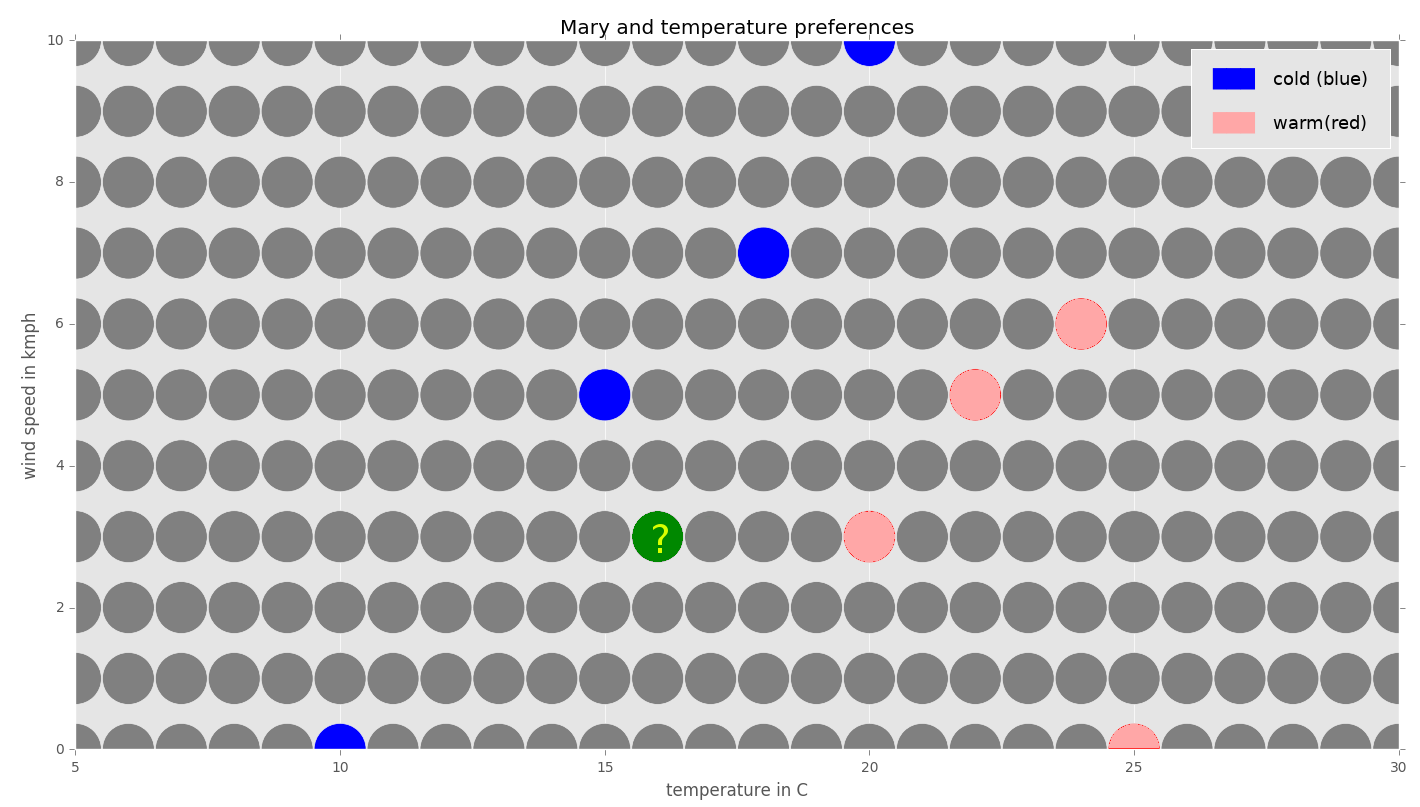For simplicity, we will use a Manhattan metric to measure the distance between the neighbors on the grid. The Manhattan distance dMan of the neighbor N1=(x1,y1) from the neighbor N2=(x2,y2) is defined as dMan=|x1-x2|+|y1-y2|.

Let's label the grid with distances around the neighbors to see which neighbor with a known class is closest to the point we would like to classify: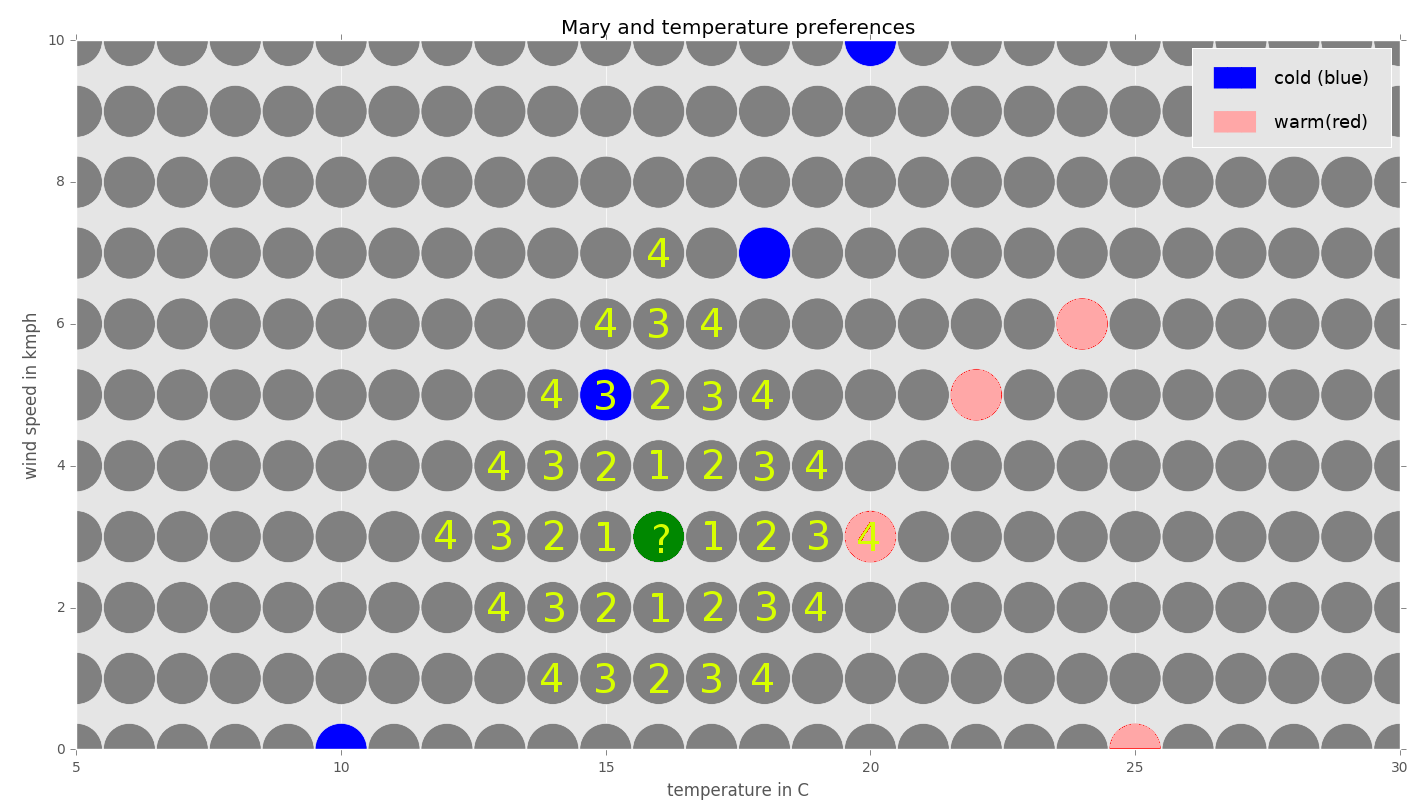We can see that the closest neighbor with a known class is the one with a temperature of 15°C (blue) and a wind speed of 5 km/h. Its distance from the point in question is three units. Its class is blue (cold). The closest red (warm) neighbour is at a distance of four units from the point in question. Since we are using the 1-nearest neighbor algorithm, we just look at the closest neighbor and, therefore, the class of the point in question should be blue (cold).

By applying this procedure to every data point, we can complete the graph, as follows: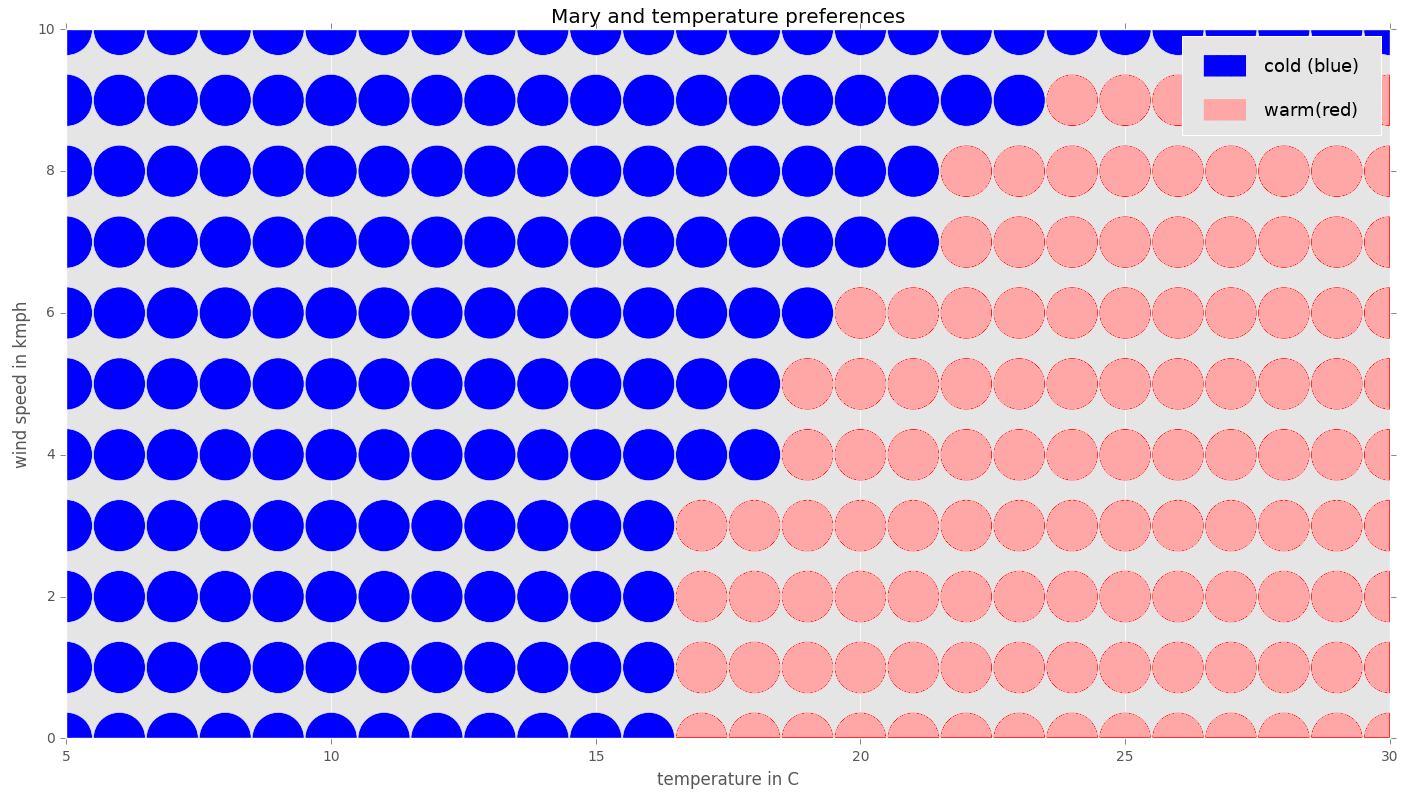Note that, sometimes, a data point might be the same distance away from two known classes: for example, 20°C and 6 km/h. In such situations, we could prefer one class over the other, or ignore these boundary cases. The actual result depends on the specific implementation of an algorithm.

## Implementation of the k-nearest neighbors algorithm

Now, we will implement the k-NN algorithm in Python to find Mary's temperature preference. At the end of this section, we will also implement the visualization of the data produced in the previous section, that is, Mary and her temperature preferences. The full, compilable code, with the input files, can be found in the source code provided with this book. The most important parts have been extracted and presented here:

```# source_code/1/mary_and_temperature_preferences/knn_to_data.py
# Applies the knn algorithm to the input data.
# The input text file is assumed to be of the format with one line per
# every data entry consisting of the temperature in degrees Celsius,
# wind speed and then the classification cold/warm.

import sys
sys.path.append('..')
sys.path.append('../../common')
import knn # noqa
import common # noqa

# Program start
# E.g. "mary_and_temperature_preferences.data"
input_file = sys.argv
# E.g. "mary_and_temperature_preferences_completed.data"
output_file = sys.argv
k = int(sys.argv)
x_from = int(sys.argv)
x_to = int(sys.argv)
y_from = int(sys.argv)
y_to = int(sys.argv)

new_data = knn.knn_to_2d_data(data, x_from, x_to, y_from, y_to, k)
common.save_3row_data_from_dic(output_file, new_data)```
```# source_code/common/common.py
# ***Library with common routines and functions***
def dic_inc(dic, key):
if key is None:
pass
if dic.get(key, None) is None:
dic[key] = 1
else:
dic[key] = dic[key] + 1
```
```# source_code/1/knn.py
# ***Library implementing knn algorithm***

def info_reset(info):
info['nbhd_count'] = 0
info['class_count'] = {}

# Find the class of a neighbor with the coordinates x,y.
# If the class is known count that neighbor.
group = data.get((x, y), None)
common.dic_inc(info['class_count'], group)
info['nbhd_count'] += int(group is not None)

# Apply knn algorithm to the 2d data using the k-nearest neighbors with
# the Manhattan distance.
# The dictionary data comes in the form with keys being 2d coordinates
# and the values being the class.
# x,y are integer coordinates for the 2d data with the range
# [x_from,x_to] x [y_from,y_to].
def knn_to_2d_data(data, x_from, x_to, y_from, y_to, k):
new_data = {}
info = {}
# Go through every point in an integer coordinate system.
for y in range(y_from, y_to + 1):
for x in range(x_from, x_to + 1):
info_reset(info)
# Count the number of neighbors for each class group for
# every distance dist starting at 0 until at least k
# neighbors with known classes are found.
for dist in range(0, x_to - x_from + y_to - y_from):
# Count all neighbors that are distanced dist from
# the point [x,y].
if dist == 0:
else:
for i in range(0, dist + 1):
info_add(info, data, x - i, y + dist - i)
info_add(info, data, x + dist - i, y - i)
for i in range(1, dist):
info_add(info, data, x + i, y + dist - i)
info_add(info, data, x - dist + i, y - i)
# There could be more than k-closest neighbors if the
# distance of more of them is the same from the point
# [x,y]. But immediately when we have at least k of
# them, we break from the loop.
if info['nbhd_count'] >= k:
break
class_max_count = None
# Choose the class with the highest count of the neighbors
# from among the k-closest neighbors.
for group, count in info['class_count'].items():
if group is not None and (class_max_count is None or
count > info['class_count'][class_max_count]):
class_max_count = group
new_data[x, y] = class_max_count
return new_data```

Input:

The preceding program will use the following file as the source of the input data. The file contains the table with the known data about Mary's temperature preferences:

```# source_code/1/mary_and_temperature_preferences/
marry_and_temperature_preferences.data
10 0 cold
25 0 warm
15 5 cold
20 3 warm
18 7 cold
20 10 cold
22 5 warm
24 6 warm```

Output:

We run the preceding implementation on the `mary_and_temperature_preferences.data` input file by using the k-NN algorithm for `k=1` neighbors. The algorithm classifies all the points with integer coordinates in the rectangle with a size of `(30-5=25) by (10-0=10)`, hence, with a size of `(25+1) * (10+1) = 286` integer points (adding one to count points on boundaries). Using the `wc` command, we find out that the output file contains exactly 286 lines—one data item per point. Using the `head` command, we display the first 10 lines from the output file:

```\$ python knn_to_data.py mary_and_temperature_preferences.data mary_and_temperature_preferences_completed.data 1 5 30 0 10

\$ wc -l mary_and_temperature_preferences_completed.data
286 mary_and_temperature_preferences_completed.data

7 3 cold
6 9 cold
12 1 cold
16 6 cold
16 9 cold
14 4 cold
13 4 cold
19 4 warm
18 4 cold
15 1 cold```

Visualization:

For the visualization depicted earlier in this chapter, the `matplotlib` library was used. A data file is loaded, and then displayed in a scatter diagram:

```# source_code/common/common.py
# returns a dictionary of 3 lists: 1st with x coordinates,
# 2nd with y coordinates, 3rd with colors with numeric values
def get_x_y_colors(data):
dic = {}
dic['x'] =  * len(data)
dic['y'] =  * len(data)
dic['colors'] =  * len(data)
for i in range(0, len(data)):
dic['x'][i] = data[i]
dic['y'][i] = data[i]
dic['colors'][i] = data[i]
return dic```
```# source_code/1/mary_and_temperature_preferences/
mary_and_temperature_preferences_draw_graph.py
import sys
sys.path.append('../../common')  # noqa
import common
import numpy as np
import matplotlib.pyplot as plt
import matplotlib.patches as mpatches
import matplotlib
matplotlib.style.use('ggplot')

data_file_name = 'mary_and_temperature_preferences_completed.data'
temp_from = 5
temp_to = 30
wind_from = 0
wind_to = 10

dtype={'names': ('temperature', 'wind', 'perception'),
'formats': ('i4', 'i4', 'S4')})

# Convert the classes to the colors to be displayed in a diagram.
for i in range(0, len(data)):
if data[i] == 'cold':
data[i] = 'blue'
elif data[i] == 'warm':
data[i] = 'red'
else:
data[i] = 'gray'
# Convert the array into the format ready for drawing functions.
data_processed = common.get_x_y_colors(data)

# Draw the graph.
plt.title('Mary and temperature preferences')
plt.xlabel('temperature in C')
plt.ylabel('wind speed in kmph')
plt.axis([temp_from, temp_to, wind_from, wind_to])
# Add legends to the graph.
blue_patch = mpatches.Patch(color='blue', label='cold')
red_patch = mpatches.Patch(color='red', label='warm')
plt.legend(handles=[blue_patch, red_patch])
plt.scatter(data_processed['x'], data_processed['y'],
c=data_processed['colors'], s= * len(data))
plt.show()```

## Map of Italy example – choosing the value of k

In our data, we are given some points (about 1 percent) from the map of Italy and its surroundings. The blue points represent water, and the green points represent land; the white points are unknown. From the partial information that we have been given, we would like to predict whether there is water or land in the white areas.

Drawing only 1% of the map data in the picture would make it almost invisible. If we were given about 33 times more data from the map of Italy and its surroundings, and drew it in the picture instead, it would look as follows: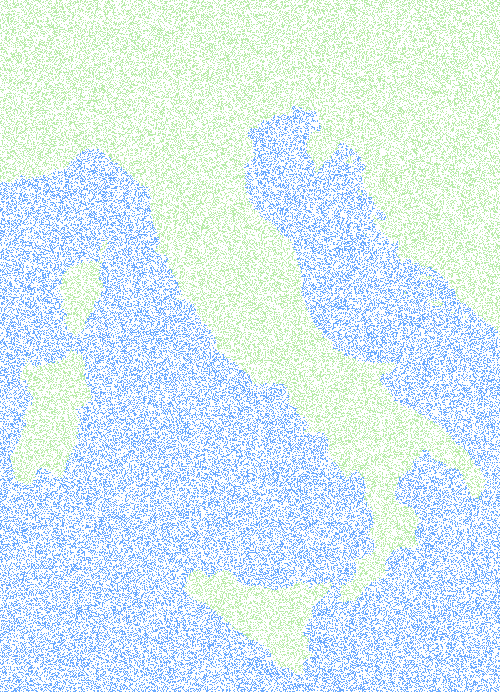### Analysis

For this problem, we will use the k-NN algorithm—k here means that we will look at k-closest neighbors. Given a white point, it will be classified as an area of water if the majority of its k-closest neighbors are in an area of water and classified as land if the majority of its k-closest neighbors are an area of land. We will use the Euclidean metric for the distance: given two points, X=[x0,x1] and Y=[y0,y1], their Euclidean distance is defined as dEuclidean = sqrt((x0-y0)2+(x1-y1)2).

The Euclidean distance is the most common metric. Given two points on a piece of paper, their Euclidean distance is just the length between the two points, as measured by a ruler, as shown in the following diagram: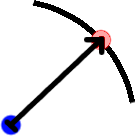To apply the k-NN algorithm to an incomplete map, we have to choose the value of k. Since the resulting class of a point is the class of the majority of the k-closest neighbors of that point, k should be odd. Let's apply this algorithm to the values of k=1,3,5,7,9.

Applying this algorithm to every white point on the incomplete map will result in the following complete maps: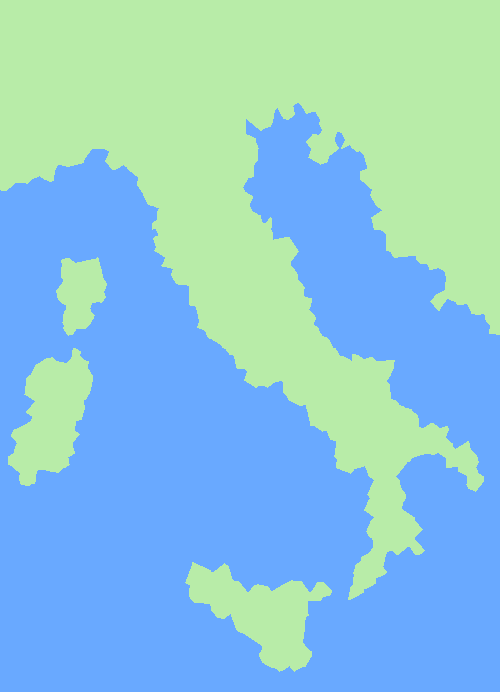k=1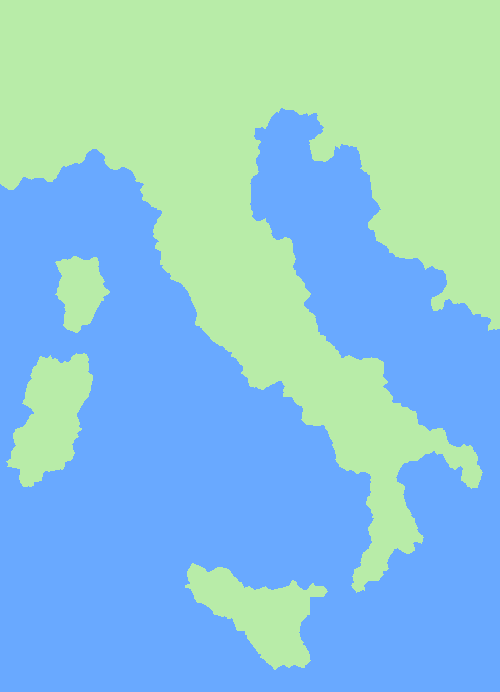k=3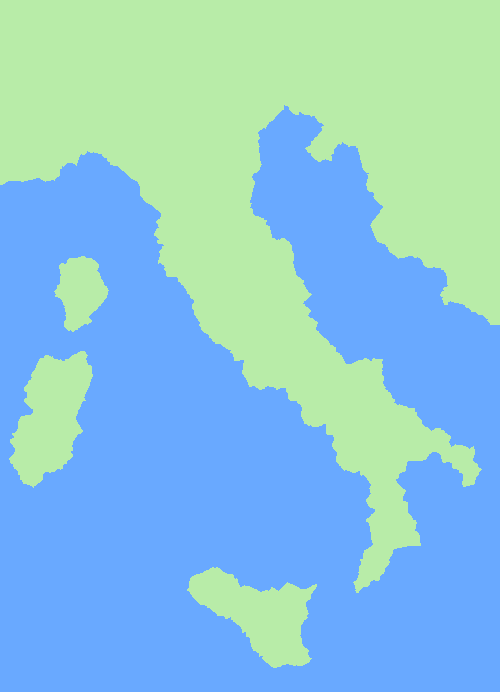k=5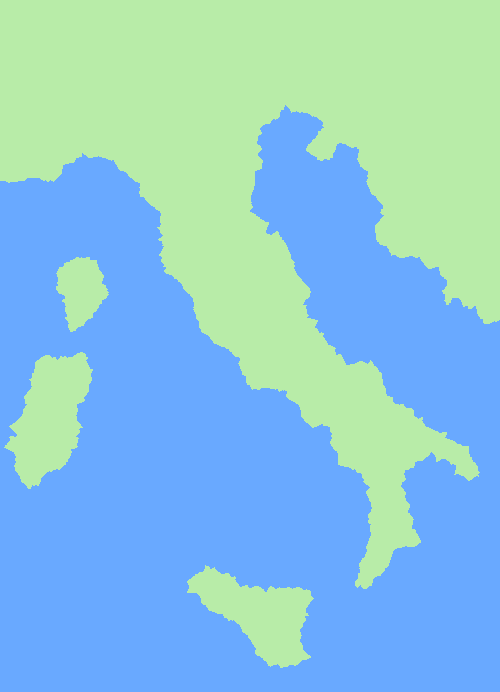k=7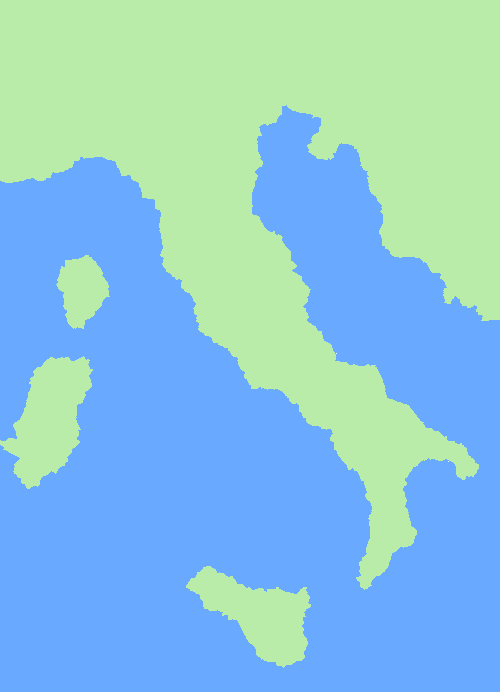k=9

As you may have noticed, the highest value of k results in a complete map with smoother boundaries. An actual complete map of Italy is shown here: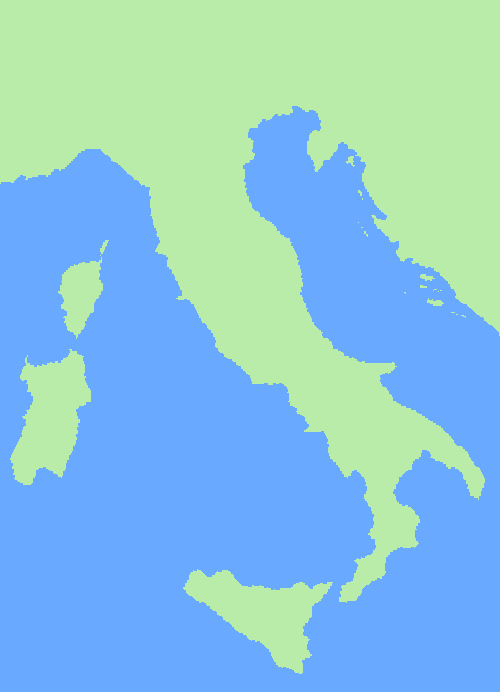We can use this real, complete map to calculate the percentage of incorrectly classified points for the various values of k to determine the accuracy of the k-NN algorithm for different values of k:

 k Precentage of incorrectly classified points 1 2.97 3 3.24 5 3.29 7 3.40 9 3.57

Thus, for this particular type of classification problem, the k-NN algorithm achieves the highest accuracy (least error rate) for k=1.

However, in real life, the problem is that we wouldn't usually have complete data or a solution. In such scenarios, we need to choose a value of k that is appropriate to the data that is partially available. For this, consultProblem 4 at the end of this chapter.

## House ownership – data rescaling

For each person, we are given their age, yearly income, and whether or not they own a house:

 Age Annual income in USD House ownership status 23 50,000 Non-owner 37 34,000 Non-owner 48 40,000 Owner 52 30,000 Non-owner 28 95,000 Owner 25 78,000 Non-owner 35 130,000 Owner 32 105,000 Owner 20 100,000 Non-owner 40 60,000 Owner 50 80,000 Peter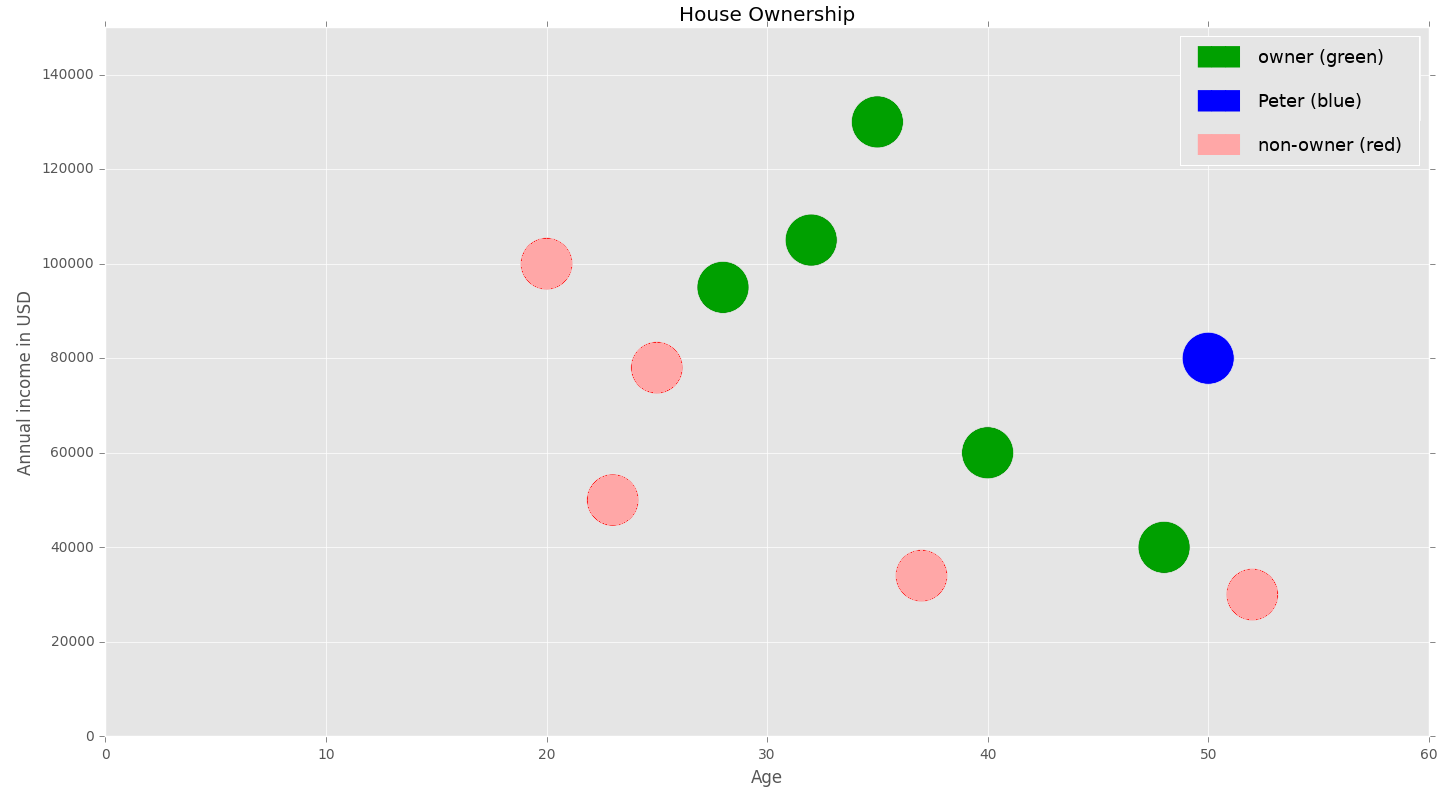House ownership and annual income

The aim is to predict whether Peter, aged 50, with an income of \$80,000 per year, owns a house and could be a potential customer for our insurance company.

### Analysis

In this case, we could try to apply the 1-NN algorithm. However, we should be careful about how we measure the distances between the data points, since the income range is much wider than the age range. Income levels of USD 115 k and USD 116 k are USD 1,000 apart. The two data points for these incomes would be very far apart. However, relative to each other, the difference between these data points isn't actually that big. Because we consider both measures (age and yearly income) to be about as important as each other, we would scale both from 0 to 1 according to the following formula:In our particular case, this reduces to the following:After scaling, we get the following data:

 Age Scaled age Annual income in USD Scaled annual income House ownership status 23 0.09375 50,000 0.2 Non-owner 37 0.53125 34,000 0.04 Non-owner 48 0.875 40,000 0.1 Owner 52 1 30,000 0 Non-owner 28 0.25 95,000 0.65 Owner 25 0.15625 78,000 0.48 Non-owner 35 0.46875 130,000 1 Owner 32 0.375 105,000 0.75 Owner 20 0 100,000 0.7 Non-owner 40 0.625 60,000 0.3 Owner 50 0.9375 80,000 0.5 ?

Now, if we apply the 1-NN algorithm with the Euclidean metric, we will find out that Peter more than likely owns a house. Note that, without rescaling, the algorithm would yield a different result. Refer to Exercise 1.5 for more information.

## Text classification – using non-Euclidean distances

We are given the following word counts relating to the keywords algorithm and computer, for documents of the classes, in the informatics and mathematics subject classifications:

 Algorithm words per 1,000 Computer words per 1,000 Subject classification 153 150 Informatics 105 97 Informatics 75 125 Informatics 81 84 Informatics 73 77 Informatics 90 63 Informatics 20 0 Mathematics 33 0 Mathematics 105 10 Mathematics 2 0 Mathematics 84 2 Mathematics 12 0 Mathematics 41 42 ?

Those documents having a high incidence of the words algorithm and computer are in the `informatics` class. The `mathematics`class happens to contain documents with a high incidence of the word algorithm in some cases, for example, a document concerned with the Euclidean algorithm from the field of number theory. But, since the `mathematics` class tends to be applied less than `informatics` in the area of algorithms, the word computer comes up less frequently in the documents.

We would like to classify a document that has 41 instances of the word algorithm per 1,000 words, and 42 instances of the word computer per 1,000 words: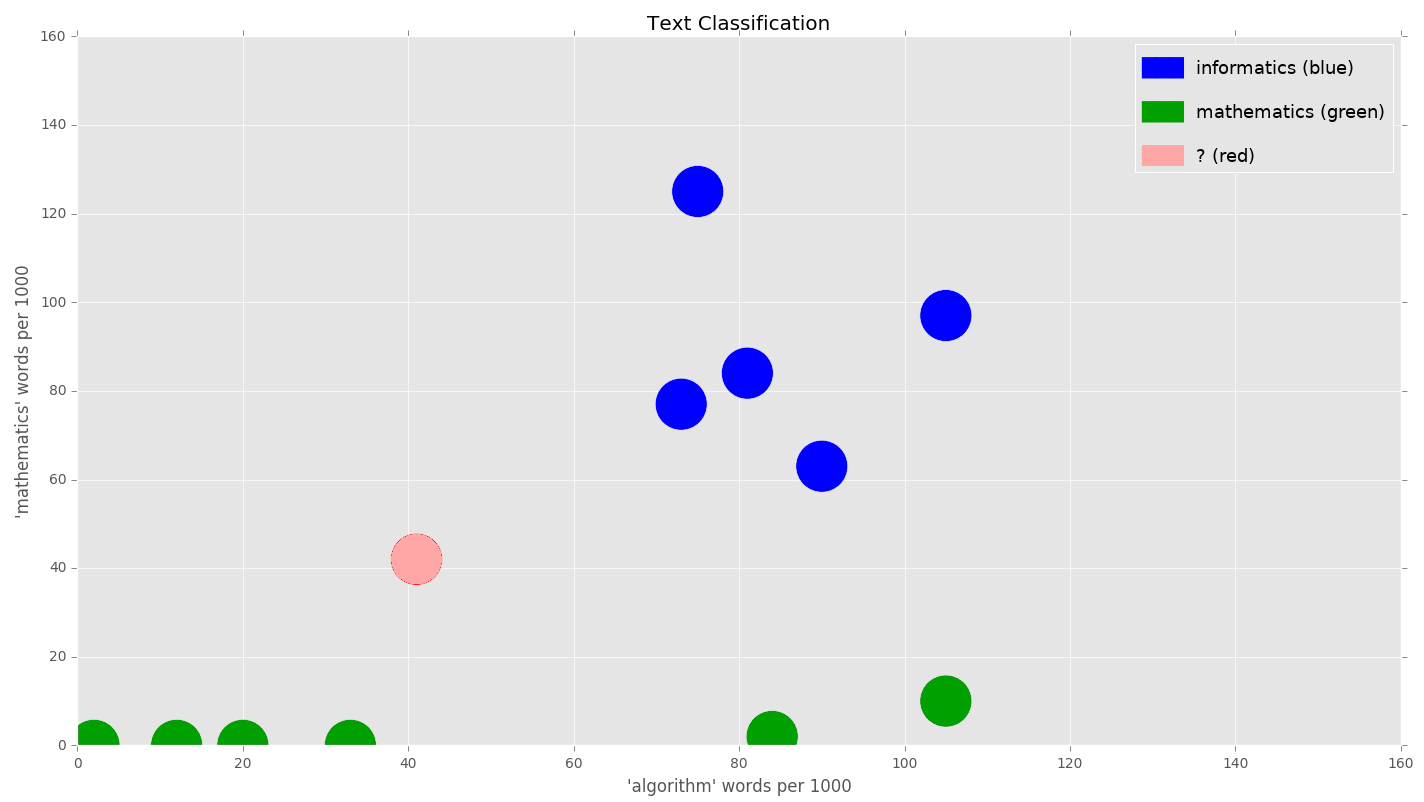### Analysis

Using, for example, the 1-NN algorithm and the Manhattan or Euclidean distance would result in the document in question being assigned to the `mathematics`class. However, intuitively, we should instead use a different metric to measure the distance, as the document in question has a much higher incidence of the word computer than other known documents in the class of `mathematics`.

Another candidate metric for this problem is a metric that would measure the proportion of the for the words or the angle between the instances in documents. Instead of the angle, you could take the cosine of the angle, cos(θ), and then use the well-known dot product formula to calculate cos(θ).

Let's use a=(ax,ay), b=(bx,by). Use the following formula:This will derive the following:Using the cosine distance metric, you could classify the document in question to the `informatics` class: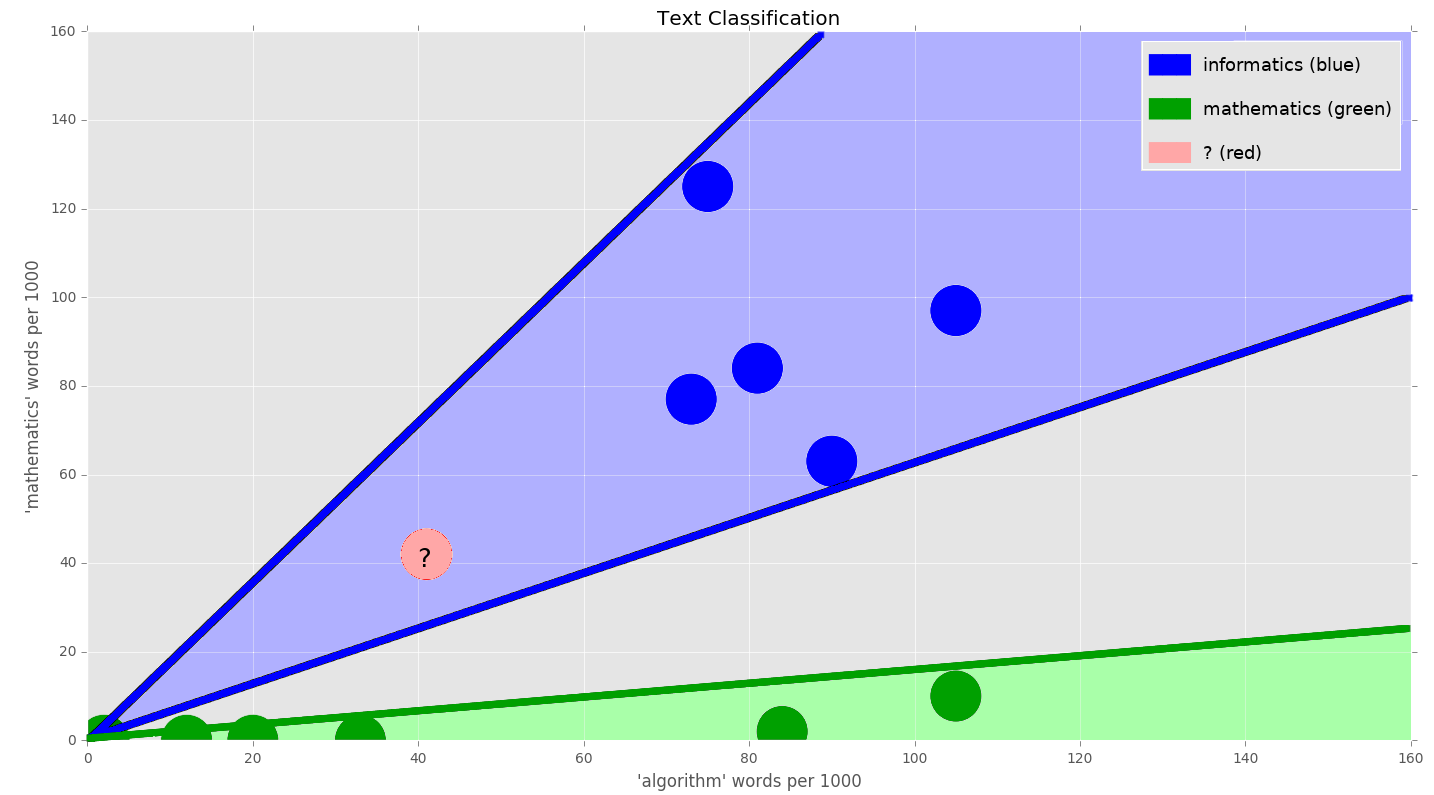## Text classification – k-NN in higher dimensions

Suppose we are given documents and would like to classify other documents based on their word frequency counts. For example, the 120 most frequently occurring words found in the Project Gutenberg e-book of the King James Bible are as follows: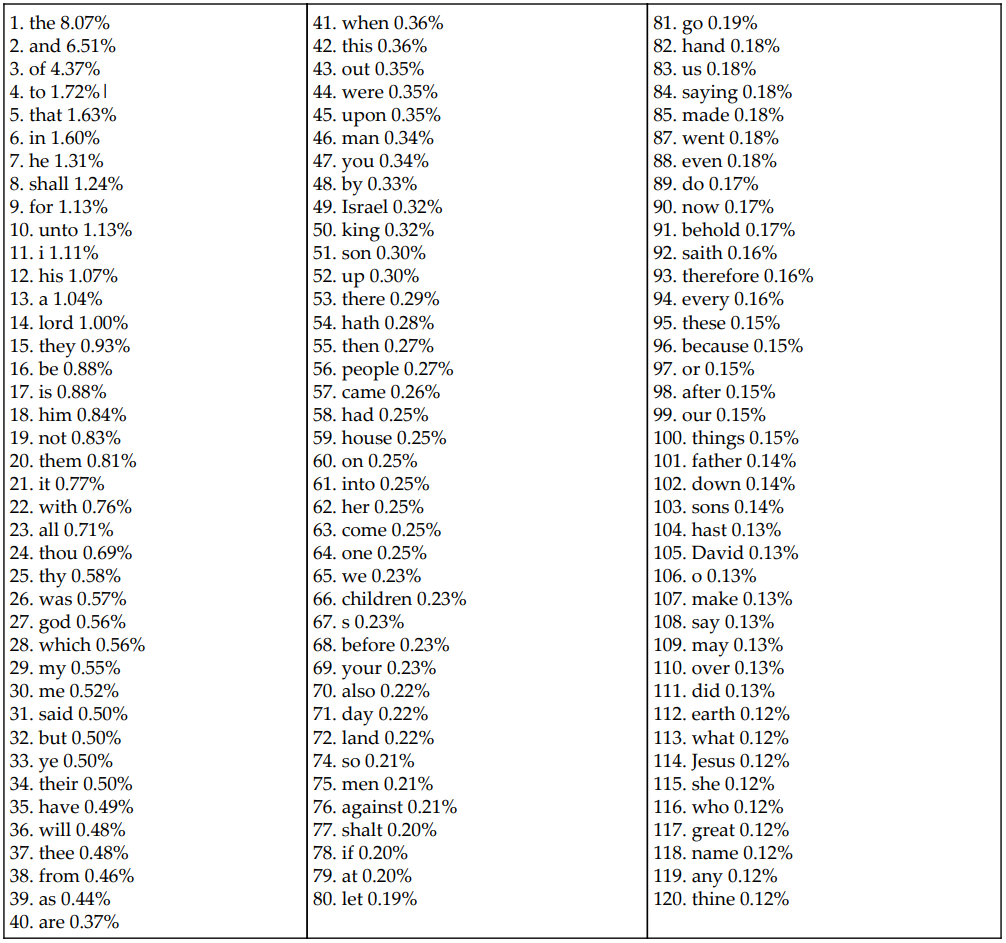The task is to design a metric that, given the word frequencies for each document, would accurately determine how semantically close those documents are. Consequently, such a metric could be used by the k-NN algorithm to classify the unknown instances in the new documents based on the existing documents.

### Analysis

Suppose that we consider, for example, N most frequent words in our corpus of documents. Then, we count the word frequencies for each of the N words in a given document and put them in an N-dimensional vector that will represent that document. Then, we define a distance between two documents to be the distance (for example, Euclidean) between the two-word frequency vectors of those documents.

The problem with this solution is that only certain words represent the actual content of the book, and others need to be present in the text because of grammar rules or their general basic meaning. For example, out of the 120 most frequently encountered words in the Bible, each word is of different importance. In the following table, we have highlighted the words that have both a high frequency in the Bible and an important meaning:

 lord - used 1.00%god - 0.56% Israel - 0.32%king - 0.32% David - 0.13%Jesus - 0.12%

These words are less likely to be present in mathematical texts, for example, but more likely to be present in texts concerned with religion or Christianity.

However, if we just look at the six most frequent words in the Bible, they happen to be less useful with regard to detecting the meaning of the text:

 the - 8.07%and - 6.51% of - 4.37%to - 1.72% that - 1.63%in - 1.60%

Texts concerned with mathematics, literature, and other subjects will have similar frequencies for these words. Differences may result mostly from the writing style.

Therefore, to determine a similarity distance between two documents, we only need to look at the frequency counts of the important words. Some words are less important—these dimensions are better reduced, as their inclusion can lead to misinterpretation of the results in the end. Thus, what we are left to do is choose the words (dimensions) that are important to classify the documents in our corpus. For this, consultProblem 6.

## Summary

In this chapter, we learned that the k-nearest neighbor algorithm is a classification algorithm that assigns the majority class among the k-nearest neighbors to a given data point. The distance between two points is measured by a metric. We covered examples of distances, including the Euclidean distance, Manhattan distance, tangential distance, and cosine distance. We also discussed how experiments with various parameters and cross-validation can help to establish which parameter, k, and which metric should be used.

We also learned that the dimensionality and position of a data point are determined by its qualities. A large number of dimensions can result in low accuracy of the k-NN algorithm. Reducing the dimensions of qualities of lesser importance can increase accuracy. Similarly, to increase accuracy further, distances for each dimension should be scaled according to the importance of the quality of that dimension.

In the next chapter, we will look at the Naive Bayes algorithm, which classifies an element based on probabilistic methods using Bayes' theorem.

## Problems

In this section, we will discuss the following problems:

• Mary and her temperature preference problems
• Map of Italy – choosing the value of k
• House ownership

In order to learn from the material from this chapter in the best way possible, please analyze these problems on your own first before looking at the Analysis section at the end of this chapter.

### Mary and her temperature preference problems

Problem 1: Imagine that you know that your friend Mary feels cold when it is -50°C, but she feels warm when it is 20°C. What would the 1-NN algorithm say about Mary? Would she feel warm or cold at temperatures of 22, 15, and -10? Do you think that the algorithm predicted Mary's body's perception of the temperature correctly? If not, please give your reasons and suggest why the algorithm did not give appropriate results and what would need to be improved for the algorithm to make a better classification.

Problem 2: Do you think that the 1-NN algorithm would yield better results than using the k-NN algorithm for k>1?

Problem 3: We collected more data and found out that Mary feels warmat 17°C, but cold at 18°C. Using our own common sense, Mary should feel warmer when the temperature is higher. Can you explain the possible cause of the discrepancies in the data? How could we improve the analysis of our data? Should we also collect some non-temperature data? Suppose that we have the only piece of temperature data available; do you think that the 1-NN algorithm would still yield better results with data like this? How should we choosekfor the k-NN algorithm to perform well?

### Map of Italy – choosing the value of k

Problem 4: We are given a partial map of Italy for the Map of Italy problem. However, suppose that the complete data is not available. Thus, we cannot calculate the error rate on all of the predicted points for different values of k. How should you choose the value of k for the k-NN algorithm, to complete the map of Italy with a view to maximizing its accuracy?

### House ownership

Problem 5: Using the data from the section concerned with the problem of house ownership, find the closest neighbor to Peter by using the Euclidean metric:

a) Without rescaling the data

b) By using the scaled data

Is the closest neighbor in:

a) The same as the neighbor in?

b) Which of the neighbors owns the house?

Problem 6: Suppose you would like to find books or documents in Gutenberg's corpus (www.gutenberg.org) that are similar to a selected book from the corpus (for example, the Bible) by using a certain metric and the 1-NN algorithm. How would you design a metric measuring the similarity distance between the two books?

### Analysis

Problem 1: 8°C is closer to 20°C than to -50°C. So, the algorithm would classify Mary as feeling warm at -8°C. But this is not likely to be true if we use our common sense and knowledge. In more complex examples, we may be deluded by the results of the analysis and make false conclusions due to our lack of expertise. But remember that data science makes use of substantive and expert knowledge, not only data analysis. In order to draw sound conclusions, we should have a good understanding of the problem and our data.

The algorithm further says that at 22°C, Mary should feel warm, and there is no doubt in that, as 22°C is higher than 20°C, and a human being feels warmer with a higher temperature; again, a trivial use of our knowledge. For 15°C, the algorithm would deem Mary to feel warm, but if we use our common sense, we may not be that certain of this statement.

To be able to use our algorithm to yield better results, we should collect more data. For example, if we find out that Mary feels cold at 14°C, then we have a data instance that is very close to 15° and, thus, we can guess with a higher degree of certainty that Mary would feel cold at a temperature of 15°.

Problem 2: The data we are dealing with is just one-dimensional and is also partitioned into two parts, cold and warm, with the following property: the higher the temperature, the warmer a person feels. Also, even if we know how Mary feels at the temperatures -40, -39, …, 39, and 40, we still have a very limited amount of data instances – just one for around every degree Celsius. For these reasons, it is best to just look at one closest neighbor.

Problem 3: Discrepancies in the data can be caused by inaccuracies in the tests carried out. This could be mitigated by performing more experiments.

Apart from inaccuracy, there could be other factors that influence how Mary feels: for example, the wind speed, humidity, sunshine, how warmly Mary is dressed (whether she has a coat on with jeans, or just shorts with a sleeveless top, or even a swimming suit), and whether she is wet or dry. We could add these additional dimensions (wind speed and how she is dressed) into the vectors of our data points. This would provide more, and better quality, data for the algorithm and, consequently, better results could be expected.

If we have only temperature data, but more of it (for example, 10 instances of classification for every degree Celsius), then we could increase the k value and look at more neighbors to determine the temperature more accurately. But this purely relies on the availability of the data. We could adapt the algorithm to yield the classification based on all the neighbors within a certain distance, d, rather than classifying based on the k-closest neighbors. This would make the algorithm work well in both cases when we have a lot of data within a close distance, and when we have just one data instance close to the instance that we want to classify.

Problem 4: For this purpose, you can use cross-validation (consult the Cross-validation section in Appendix A – Statistics) to determine the value of k with the highest accuracy. You could separate the available data from the partial map of Italy into learning and test data, for example, 80% of the classified pixels on the map would be given to a k-NN algorithm to complete the map. Then, the remaining 20% of the classified pixels from the partial map would be used to calculate the percentage of pixels with the correct classification according to the k-NN algorithm.

Problem 5:

a) Without data rescaling, Peter's closest neighbor has an annual income of USD 78,000 and is aged 25. This neighbor does not own a house.

b) After data rescaling, Peter's closest neighbor has an annual income of USD 60,000 and is aged 40. This neighbor owns a house.

Problem 6: To design a metric that accurately measures the similarity distance between the two documents, we need to select important words that will form the dimensions of the frequency vectors for the documents. Words that do not determine the semantic meaning of documents tend to have an approximately similar frequency count across all documents. Thus, instead, we could produce a list with the relative word frequency counts for a document. For example, we could use the following definition:Then, the document could be represented by an N-dimensional vector consisting of the word frequencies for N words with the highest relative frequency count. Such a vector will tend to consist of more important words than a vector of N words with the highest frequency count.